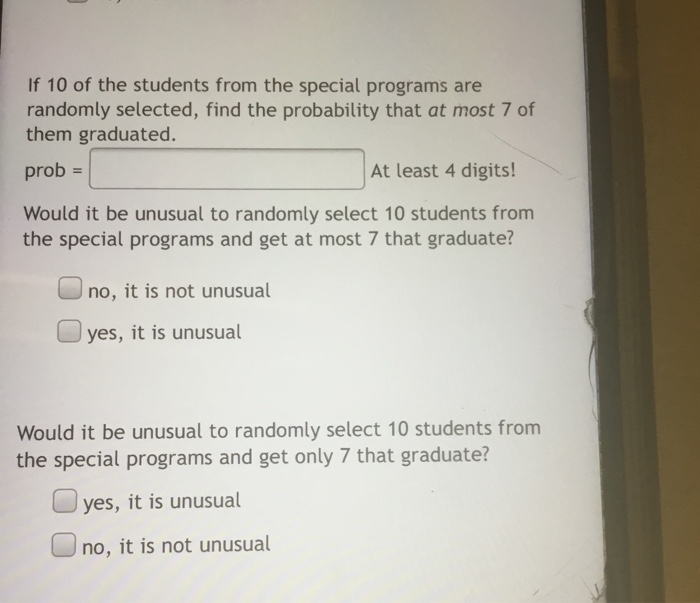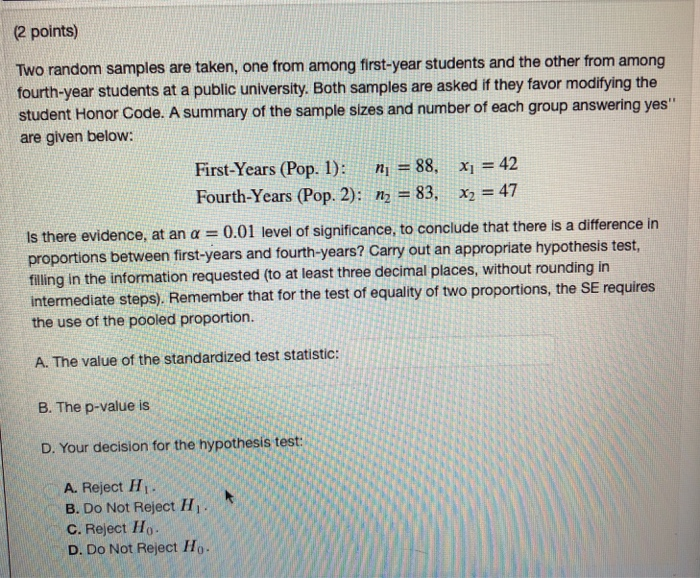# Teresa and Julia are among 10 students who have applied for a trip to Washington, D.C. Two students from the group will be selected at random for the trip. What is the probability that Teresa and Julia will be the 2 students selected?

Teresa and Julia are among 10 students who have applied for a trip to Washington, D.C. Two students from the group will be selected at random for the trip. What is the probability that Teresa and Julia will be the 2 students selected?

ReportAnswer 1

$\frac{1}{45}$

#### Explanation:

For this question, we're looking at the number of ways we can satisfy a certain condition (having Teresa and Julia be picked) over the number of ways a group of 2 students can be picked.

Since we don't care in what order people are picked, we're working with combinations, where the general formula is:

C_(n,k)=(n!)/((k!)(n-k)!) with $n = \text{population", k="picks}$

How many ways can 2 people be picked from a group of 10?

C_(10,2)=(10!)/((2!)(10-2)!)=(10!)/((2!)(8!))=(10xx9xx8!)/(2xx8!)=45#

There's only one combination that allows Teresa and Julia to be picked.

Putting it together, we have $\frac{1}{45}$

##### Add Answer of: Teresa and Julia are among 10 students who have applied for a trip to Washington, D.C. Two students from the group will be selected at random for the trip. What is the probability that Teresa and Julia will be the 2 students selected?
Similar Homework Help Questions

• ### About the group of students who are selecting 2 of their group at random to give a report, but...

About the group of students who are selecting 2 of their group at random to give a report, but assume that there are 7 males and 3 females.what is the prbability that 2 females are selected?what is the probability that 2 males are selected?

• ### Two random samples are taken, one from among UVA students and the other from among UNC...

Two random samples are taken, one from among UVA students and the other from among UNC students. Both groups are asked if academics are their top priority. A summary of the sample sizes and proportions of each group answering yes'' are given below: UVA (Pop. 1):UNC (Pop. 2):n1=80,n2=99,p^1=0.736p^2=0.602 Find a 97.9% confidence interval for the difference p1−p2 of the population proportions. (Give your answer as two values inside parentheses, separated by a comma, and rounded to at least three decimal...

• ### Two random samples are taken, one from among UVA students and the other from among UNC...

Two random samples are taken, one from among UVA students and the other from among UNC students. Both groups are asked if academics are their top priority. A summary of the sample sizes and proportions of each group answering yes'' are given below: UVA (Pop. 1): UNC (Pop. 2): n 1 =84, n 2 =81, p ^ 1 =0.749 p ^ 2 =0.555 UVA (Pop. 1):n1=84,p^1=0.749UNC (Pop. 2):n2=81,p^2=0.555 Find a 93.3% confidence interval for the difference p 1 − p...

• ### If 10 of the students from the special programs are randomly selected, find the probability that...If 10 of the students from the special programs are randomly selected, find the probability that at most 7 of them graduated. prob = At least 4 digits! Would it be unusual to randomly select 10 students from the special programs and get at most 7 that graduate? no, it is not unusual yes, it is unusual Would it be unusual to randomly select 10 students from the special programs and get only 7 that graduate? yes, it is unusual...

• ### (2 points) Two random samples are taken, one from among first-year students and the other from...(2 points) Two random samples are taken, one from among first-year students and the other from among fourth-year students at a public university. Both samples are asked if they favor modifiying the student Honor Code. A summary of the sample sizes and number of each group answering yes" are given below: First-Years (Pop. 1): n 88. x42 Fourth-Years (Pop. 2): n2-83, x2 = 47 idence, at an α-0.01 level of significance, to conclude that there is a difference in between...

• ### Two random samples are taken, one from among first-year students and the other from among fourth-year...

Two random samples are taken, one from among first-year students and the other from among fourth-year students at a public university. Both samples are asked if they favor modifying the student Honor Code. A summary of the sample sizes and number of each group answering yes'' are given below: First-Years (Pop. 1):Fourth-Years (Pop. 2):?1=88,?2=98,?1=46?2=53 Is there evidence, at an ?=0.04 level of significance, to conclude that there is a difference in proportions between first-years and fourth-years? Carry out an appropriate...

• ### Two random samples are taken, one from among first-year students and the other from among fourth-year...

Two random samples are taken, one from among first-year students and the other from among fourth-year students at a public university. Both samples are asked if they favor modifying the student Honor Code. A summary of the sample sizes and number of each group answering yes'' are given below: First-Years (Pop. 1):Fourth-Years (Pop. 2):n1=80,n2=87,x1=35x2=48 Is there evidence, at an α=0.03 level of significance, to conclude that there is a difference in proportions between first-years and fourth-years? Carry out an appropriate...

• ### Two random samples are taken, one from among first-year students and the other from among fourth-year...

Two random samples are taken, one from among first-year students and the other from among fourth-year students at a public university. Both samples are asked if they favor modifying the student Honor Code. A summary of the sample sizes and number of each group answering yes'' are given below: First-Years (Pop. 1): Fourth-Years (Pop. 2): n 1 =84, n 2 =87, x 1 =34 x 2 =37 First-Years (Pop. 1):n1=84,x1=34Fourth-Years (Pop. 2):n2=87,x2=37 Is there evidence, at an α=0.055 α=0.055 level...

• ### Three cards are selected at random from a group of 7. Two of the cards have been marked with winning numbers. What is the probability that none of the 3 cards will have a winning number?

Free Homework App